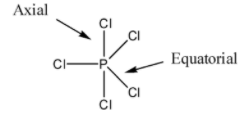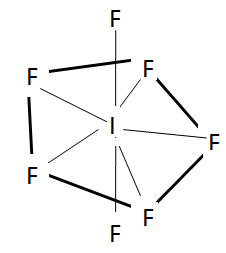Courses
Courses for Kids
Free study material
Free LIVE classes
MoreLIVE
Join Vedantu’s FREE Mastercalss

# Select the correct statement. (A) The S-F bond length is longer in $S{{F}_{6}}$ compared to that in $S{{F}_{2}}$ (B) In $PC{{l}_{5}}$ axial bonds are smaller than equatorial bonds.(C) In $I{{F}_{7}}$ axial bonds are longer than that of equatorial bonds(D) All are correct.Verified
334.5k+ views
Hint: Bond length is the distance between the nuclei of the two atoms taking part in the bond formation. The bonds that make an approximate ${{90}^{\circ }}$ angle with the plane of the atoms are called axial bonds.

We know that the stronger the force of attraction in between the bonding atoms, the smaller is the length of the bond. Now, we will analyse all options to check whether they are true or not.
So, first of all we will analyse option (A).
The bond lengths and angles in $S{{F}_{2}}$ is 159 pm and 98.2$^{\circ }$. On the other hand, $S{{F}_{6}}$ has 156pm and 90$^{\circ }$. The $S{{F}_{2}}$ bonds are actually longer than the $S{{F}_{6}}$ bonds, which is totally opposite to the more common trend in which hyper valent compounds usually have weaker and longer bonds.
So, we should note that the statement in option A is a wrong statement.

Now, we will check the statement of option (B).
So, first of all, note that in $PC{{l}_{5}}$, there are three equatorial bonds and two axial bonds. The axial bonds are normally longer than equatorial bonds because of greater repulsion from equatorial bonds. We should know that two axial bonds are at perpendicular to three equatorial bonds. So axial bonds are nearer to equatorial bonds and this causes greater repulsion resulting in elongation of bond length.So, by observing the above structure and discussions we can say that the axial bonds are longer than the equatorial bonds due to repulsion between axial and equatorial bonds. So, the statement present in option B is also wrong.

Now we will check the statement in option C. We should note that in $I{{F}_{7}}$ , it has actually 7 bond pairs. This makes it a pentagonal bipyramidal which has the axial bonds longer than the equatorial ones. The reason is the same as in the case of $PC{{l}_{5}}$ due to repulsion with equatorial bonds.
The structure of this molecule is shown here.So, from this we can say that option C is the correct statement.

Note: Do not get confused between the axial and equatorial bonds. Remember that repulsion between them is taken in consideration in order to compare their bond lengths as well as bond strength. Remember that identifying the geometry of the molecule is necessary in order to answer these types of questions.
Last updated date: 27th Sep 2023
Total views: 334.5k
Views today: 4.34k